Simulated datasets used in Baudry et al. (2010) to illustrate the proposed mixture components combining method for clustering.

Please see the cited article for a detailed presentation of these datasets. The data frame with name exN.M is presented in Section N.M in the paper.

Test1D (not in the article) has been simulated from a Gaussian mixture distribution in R.

ex4.1 and ex4.2 have been simulated from a Gaussian mixture distribution in R^2.

ex4.3 has been simulated from a mixture of a uniform distribution on a square and a spherical Gaussian distribution in R^2.

ex4.4.1 has been simulated from a Gaussian mixture model in R^2

ex4.4.2 has been simulated from a mixture of two uniform distributions in R^3.

data(Baudry_etal_2010_JCGS_examples)

Format

ex4.1 is a data frame with 600 observations on 2 real variables.

ex4.2 is a data frame with 600 observations on 2 real variables.

ex4.3 is a data frame with 200 observations on 2 real variables.

ex4.4.1 is a data frame with 800 observations on 2 real variables.

ex4.4.2 is a data frame with 300 observations on 3 real variables.

Test1D is a data frame with 200 observations on 1 real variable.

Examples

# \donttest{
data(Baudry_etal_2010_JCGS_examples)

output <- clustCombi(data = ex4.4.1)
output # is of class clustCombi
#> 'clustCombi' object:
#>  Mclust model: (VVI,4)
#>  Available object components: classification combiM combiz MclustOutput
#>  Combining matrix (K+1 classes -> K classes): <object_name>$combiM[[K]] #> Classification for K classes: <object_name>$classification[[K]]

# plots the hierarchy of combined solutions, then some "entropy plots" which
# may help one to select the number of classes
plot(output)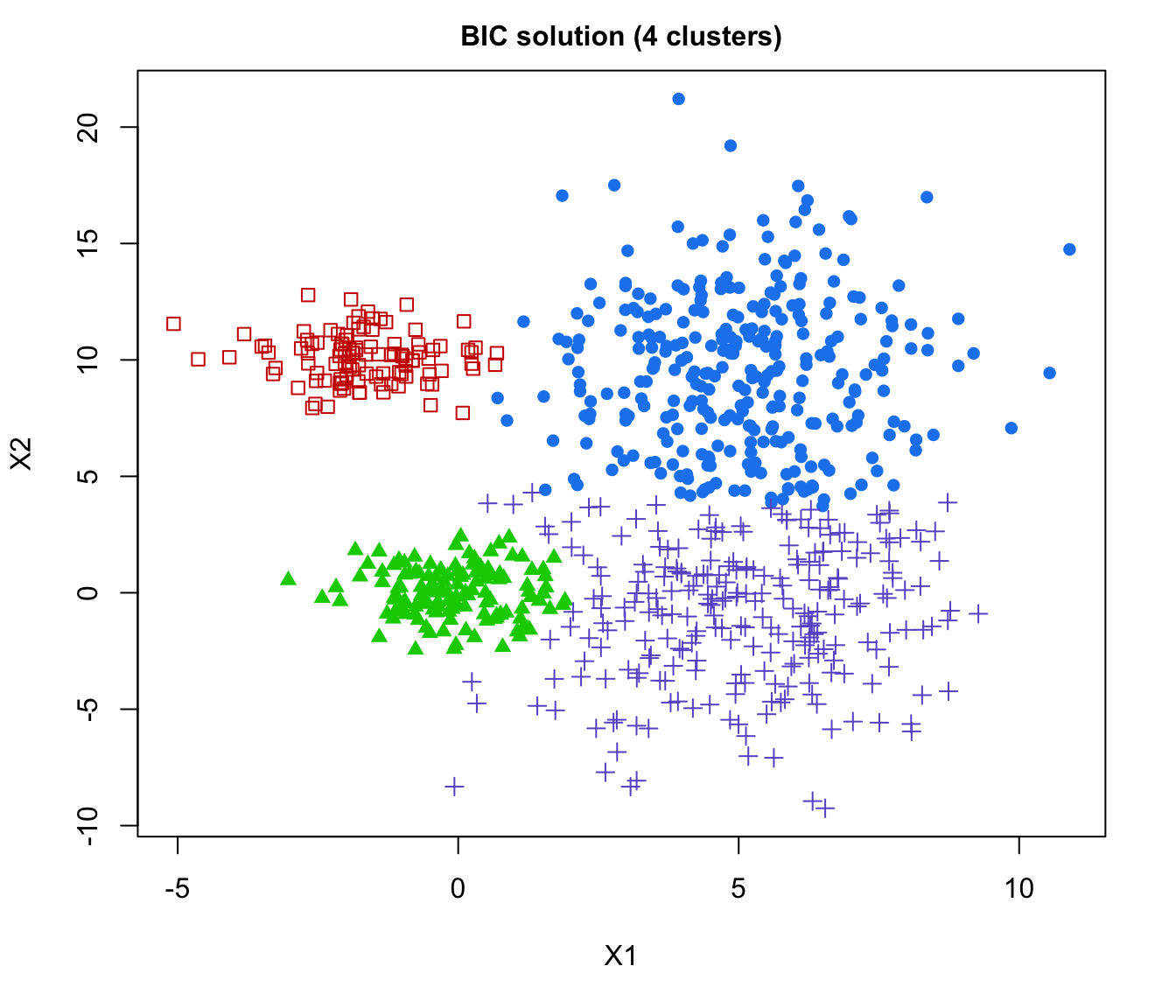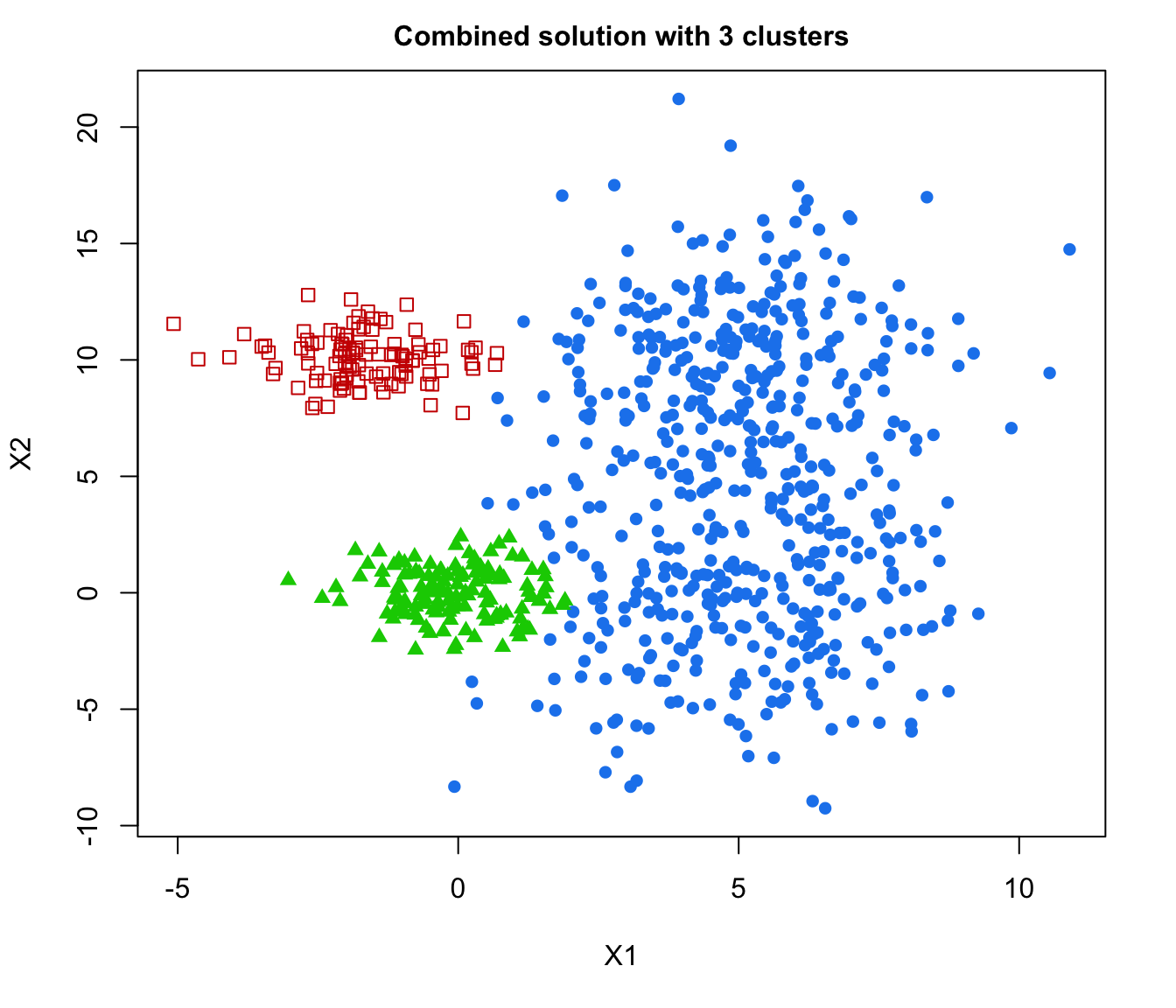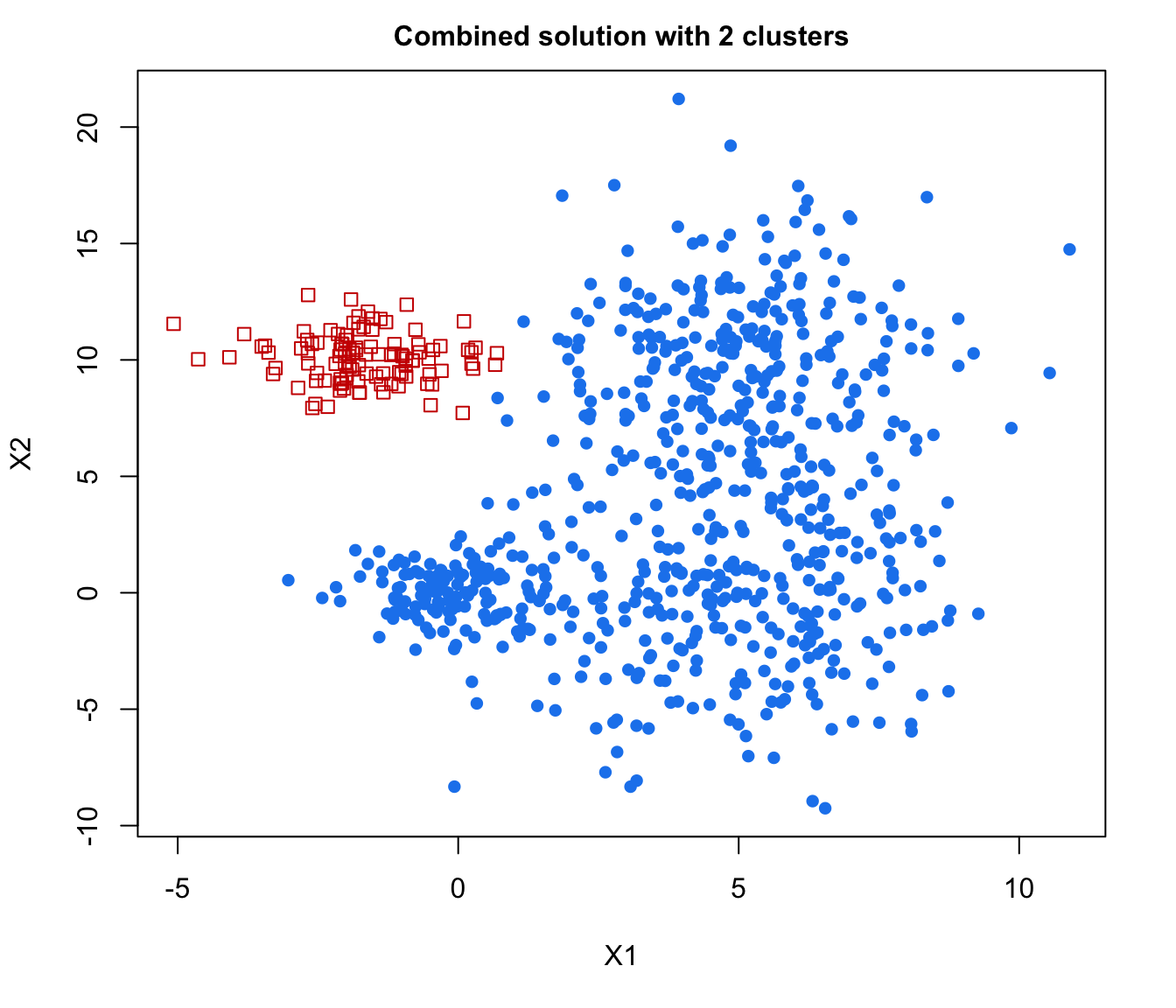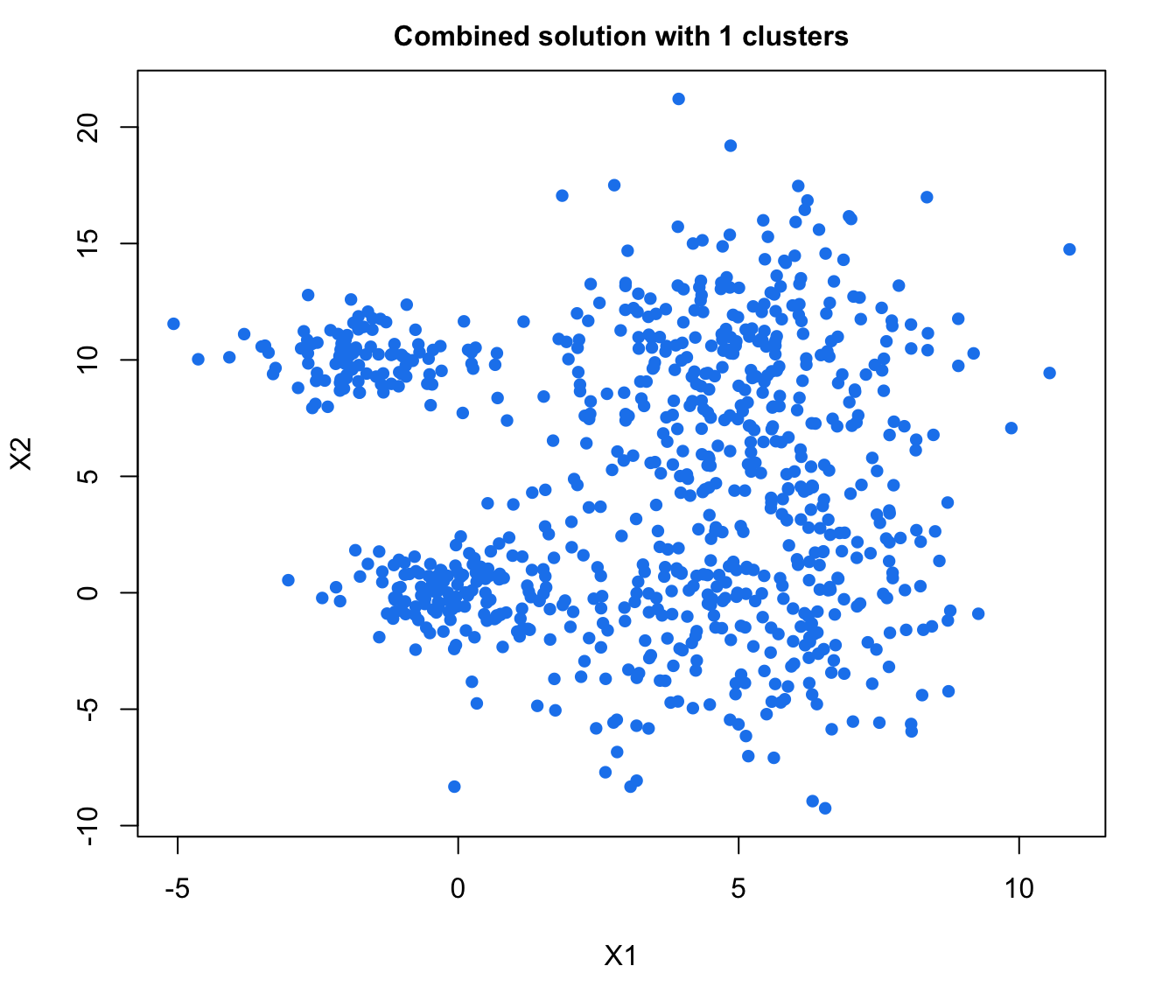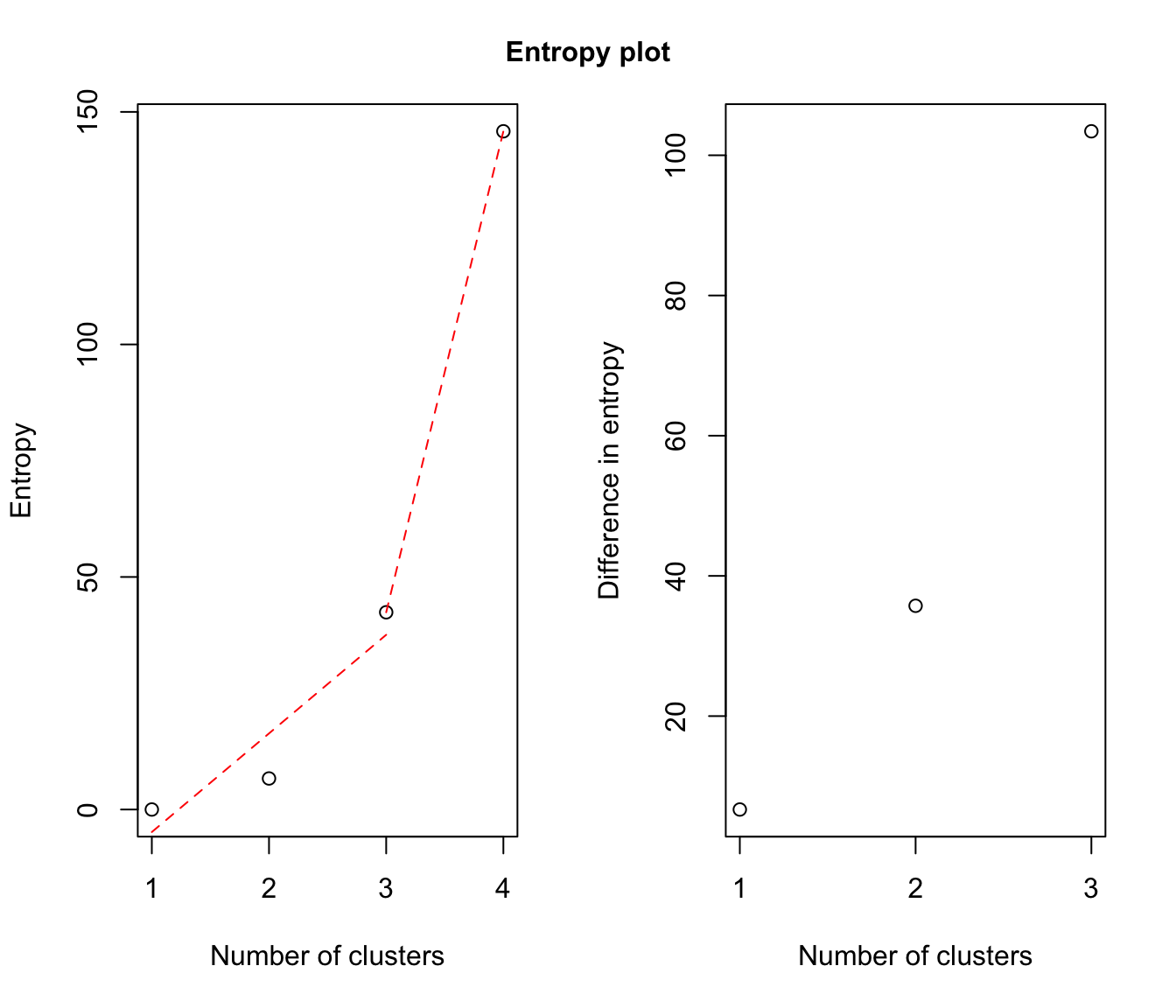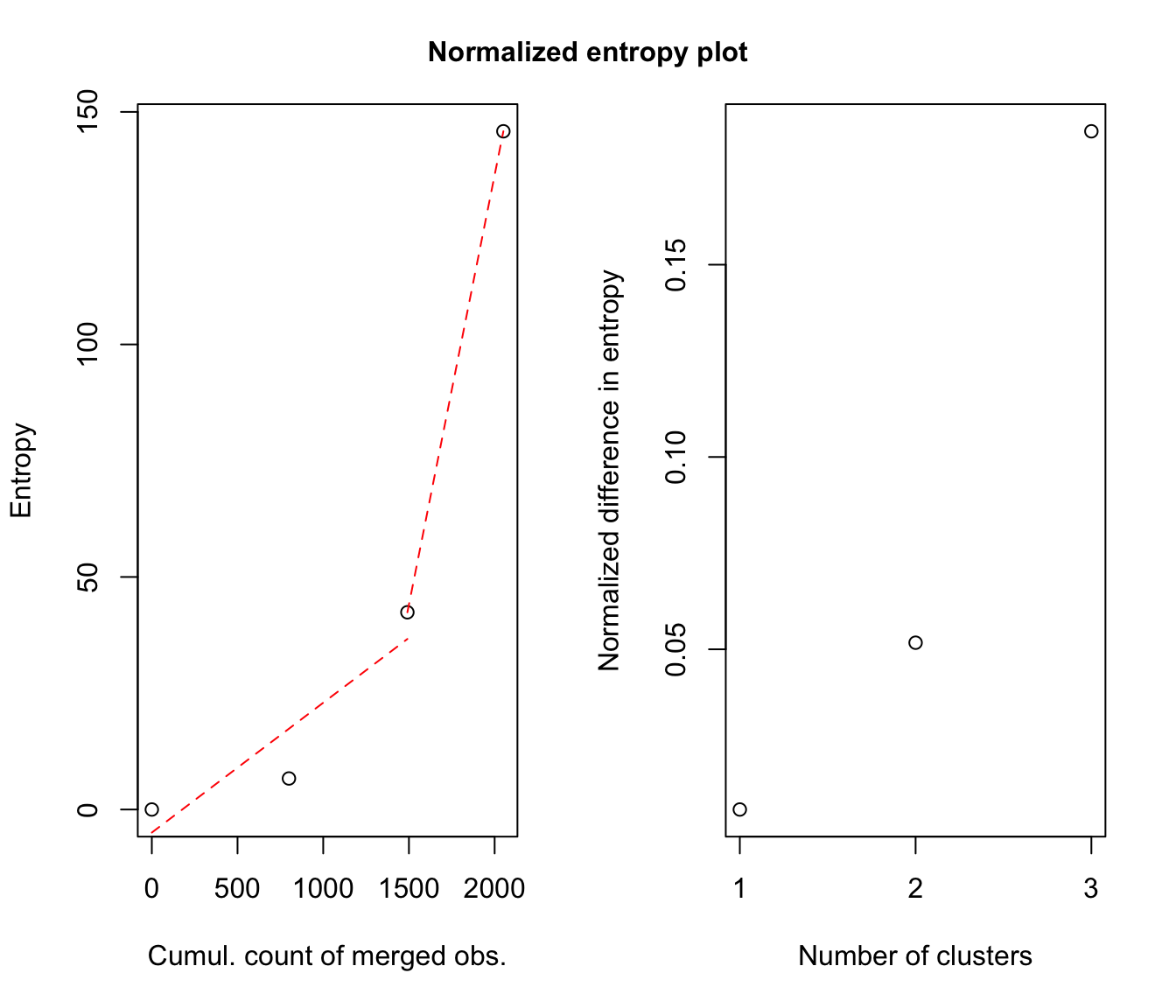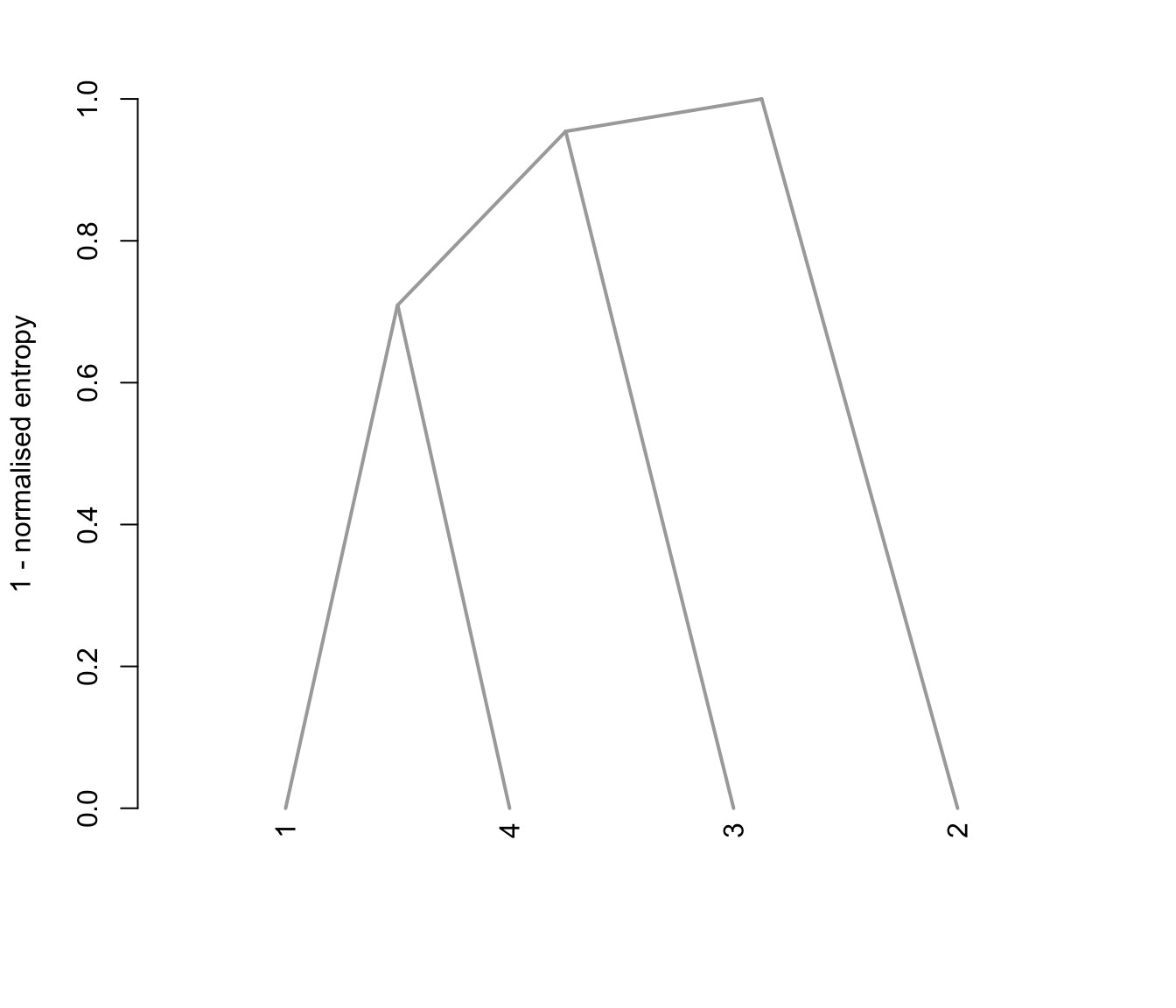# }